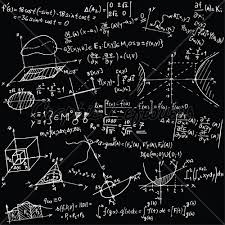# Find unknown

Find unknown numerator:

4/8 + _/8 = 1

Correct result:

n =  4

#### Solution:

4/8 + n/8 = 1

n = 4

Our simple equation calculator calculates it.We would be pleased if you find an error in the word problem, spelling mistakes, or inaccuracies and send it to us. Thank you!Tips to related online calculators
Need help calculate sum, simplify or multiply fractions? Try our fraction calculator.
Do you have a linear equation or system of equations and looking for its solution? Or do you have quadratic equation?

## Next similar math problems:

• NormThree workers planted 3555 seedlings of tomatoes in one dey. First worked at the standard norm, the second planted 120 seedlings more and the third 135 seedlings more than the first worker. How many seedlings were standard norm?
• Dropped sheetsThree consecutive sheets dropped from the book. The sum of the numbers on the pages of the dropped sheets is 273. What number has the last page of the dropped sheets?
• Write 2Write 791 thousandths as fraction in expanded form.
• Simple equation 9Solve the following equation: -8y+5=-9y+9
• Simple equationSolve for x: 3(x + 2) = x - 18
• Unknown number 11That number increased by three equals three times itself?
• Grandmother and grandfatherGrandmother baked cakes. Grandfather ate half, then quarter of the rest ate Peter and Paul ate half of rest. For parents left 6 cakes. How many cakes maked the grandmother?
• Missing numberBlank +1/6 =3/2 find the missing number
• Trees3/5 trees are apples, cherries are 1/3. 5 trees are pear. How many is the total number of trees?
• Find xSolve: if 2(x-1)=14, then x= (solve an equation with one unknown)
• ClassroomOne-eighth of 9th class was interested in studying at a grammar school, at a business academy one sixth, at secondary vocational schools quarter, to SOU one third and the remaining three students were interested in the school of art direction. How many st
• Unknown numberI think the number - its sixth is 3 smaller than its third.
• FruitsAmy bought a basket of fruits 1/5 of them were apples,1/4 were oranges, and the rest were 33 bananas. How many fruits did she buy in all?
• Unknown number 6Determine the unknown number, which is by 1.5 greater than its fourth.
• Unknown number 6Determine x if 1/6 of x is equal to 2/5 of the number 24.
• Simple equation 5Solve equation with fractions: X × 3/8 = 1/2
• Fraction + eqSolve following simple equation with fractions: -5/6(8+5b) = 75 + 5/3b